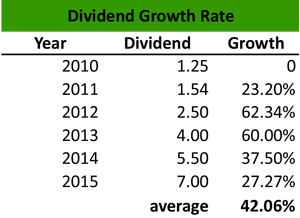# What is a Dividend Growth Rate?

Definition: The dividend growth rate is the percentage rate of growth that a dividend achieves usually on an annual basis, but it can also be addressed on a quarterly or monthly basis.

## What Does Dividend Growth Rate Mean?

What is the definition of dividend growth rate? The dividend growth rate is a component of the Dividend Discount Model (DDM) which values a stock on the basis of expected dividends, discounting them to their present value and determining if a stock trades over or under its fair value.

Investors consider firms with a solid dividend growth as safe investments that can return long-term shareholder value. Financial analysts calculate the dividend growth rate either as a single average growth rate or as a sample growth rate using the compound annual growth rate (CAGR), which calculates growth over several years.

Let’s look at an example.

## Example

Anna is a financial analyst at Telesis Securities. She wants to calculate the dividend growth rate of a construction company stock and use the rate in the dividend discount model that she prepares. The company delivers high dividends and is consistently increasing its annualized dividend each year. Anna decides to calculate the dividend growth rate both as a single average growth rate and as a compound growth rate.

The construction company has distributed the following payments over the last six years:Anna calculates the growth from one year to another, and she finds that the average dividend growth rate of the company is 42.06%.

To calculate the compound dividend growth rate, Anna divides the end dividend by the beginning dividend and raises the result to 1 over 6 which is the timeframe of her calculations. Then, she subtracts 1 from the result.

Therefore:

CAGR = (7.00 / 1.25) ^1/6 – 1 = -6.67%.

Anna is surprised to see that the CAGR calculation returns a negative value for a company that consistently delivers dividends. However, this discrepancy between the calculation of the dividend as a single average growth rate and as a compound growth rate indicates that the CAGR does not consider volatility, but also that financial analysts should always cross-check their calculations.

## Summary Definition

Define Dividend Growth Rate: The dividend growth rate is percentage increase in dividends over a period of time.